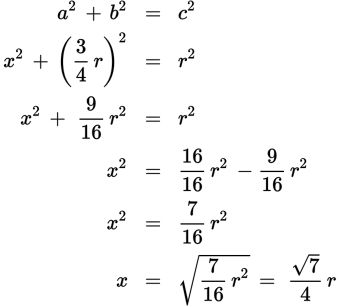# SAT Math Multiple Choice Question 284: Answer and Explanation

### Test Information

Question: 284

14.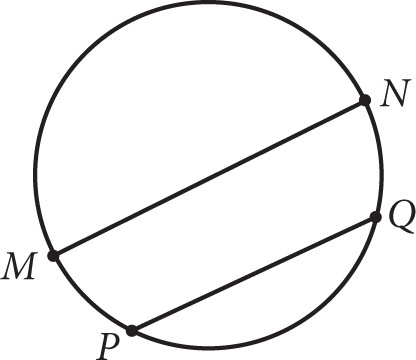The circle shown has a radius of r centimeters. If chord PQ is parallel to diameter MN, and the length of chord PQ is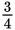of the length of the diameter, what is the distance in centimeters between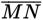and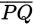in terms of r?

• A.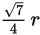• B.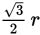• C.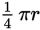• D.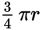Explanation:

A

Difficulty: Hard

Category: Additional Topics in Math / Geometry

Strategic Advice: Drawing in a radius or two is usually a good way to start a circle question, especially when there doesn't seem to be a lot of information given. This question asks about the distance between the chord and the diameter, so start by drawing that in. Then, see if drawing a radius will help.

Getting to the Answer: After you've drawn in anything that you think might help you answer the question, go back and label wherever possible. The radius has length r, so add that to the diagram. The chord isas long as the diameter, which means half the chord isas long as the radius, so add that to the diagram. You are looking for the distance between the chord and the diameter, so call that x.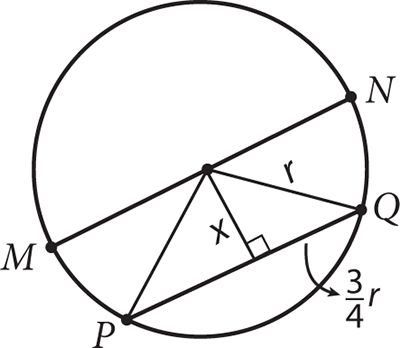You now have a right triangle with enough labels to use the Pythagorean theorem.